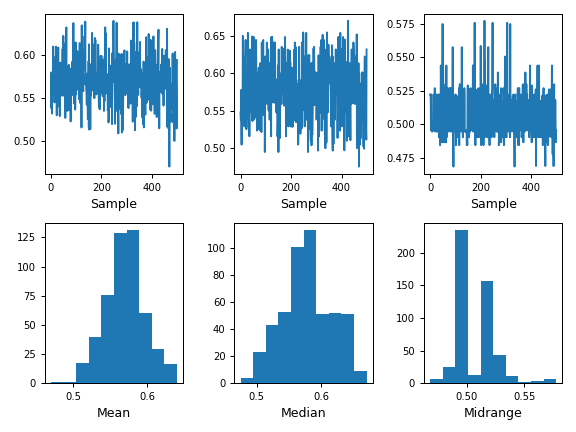# pandas.plotting.bootstrap_plot¶

`pandas.plotting.``bootstrap_plot`(series, fig=None, size=50, samples=500, **kwds)[source]

Bootstrap plot on mean, median and mid-range statistics.

The bootstrap plot is used to estimate the uncertainty of a statistic by relaying on random sampling with replacement . This function will generate bootstrapping plots for mean, median and mid-range statistics for the given number of samples of the given size.

1

“Bootstrapping (statistics)” in https://en.wikipedia.org/wiki/Bootstrapping_%28statistics%29

Parameters
seriespandas.Series

Series from where to get the samplings for the bootstrapping.

figmatplotlib.figure.Figure, default None

If given, it will use the fig reference for plotting instead of creating a new one with default parameters.

sizeint, default 50

Number of data points to consider during each sampling. It must be less than or equal to the length of the series.

samplesint, default 500

Number of times the bootstrap procedure is performed.

**kwds

Options to pass to matplotlib plotting method.

Returns
matplotlib.figure.Figure

Matplotlib figure.

`DataFrame.plot`

Basic plotting for DataFrame objects.

`Series.plot`

Basic plotting for Series objects.

Examples

This example draws a basic bootstrap plot for a Series.

```>>> s = pd.Series(np.random.uniform(size=100))
>>> pd.plotting.bootstrap_plot(s)
```